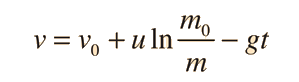# Vertical Rocket### Develop expressions

Under the influence of the rocket thrust and gravity, the rocket velocity can be calculated as follows:

For exhaust velocity u = m/s
and burn rate dM/dt = kg/s
the rocket thrust is x10^ N.
For rocket mass = kg
and fuel mass = kg, the time of burn
is seconds and the velocity at burnout is calculated fromwhich gives vburnout = m/s.
Fowles and Cassiday give as example data for a satellite launch a low orbit velocity of about 8 km/s, an initial velocity of about 0.5 km/s from the rotation of the earth near the equator, and an exhaust velocity of 2.5 km/s. You could use this data above to confirm that you could get only about 5 percent of the total initial mass into orbit. They suggest that effective ejection speeds for most rocket fuels are on the order of 2 to 4 km/s.
Index

Rocket propulsion

 HyperPhysics***** Mechanics ***** Newton's laws R Nave
Go Back